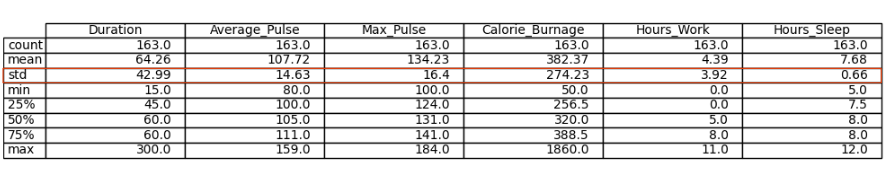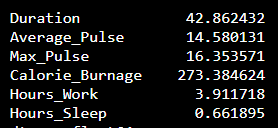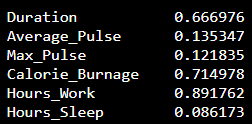# Data Science - Statistics Standard Deviation

## Standard Deviation

Standard deviation is a number that describes how spread out the observations are.A mathematical function will have difficulties in predicting precise values, if the observations are "spread". Standard deviation is a measure of uncertainty.

A low standard deviation means that most of the numbers are close to the mean (average) value.

A high standard deviation means that the values are spread out over a wider range.

Tip: Standard Deviation is often represented by the symbol Sigma: σ

We can use the `std()` function from Numpy to find the standard deviation of a variable:

### Example

import numpy as np

std = np.std(full_health_data)
print(std)
Try it Yourself »

The output:What does these numbers mean?

## Coefficient of Variation

The coefficient of variation is used to get an idea of how large the standard deviation is.

Mathematically, the coefficient of variation is defined as:

Coefficient of Variation = Standard Deviation / Mean

We can do this in Python if we proceed with the following code:

### Example

import numpy as np

cv = np.std(full_health_data) / np.mean(full_health_data)
print(cv)
Try it Yourself »

The output:We see that the variables Duration, Calorie_Burnage and Hours_Work has a high Standard Deviation compared to Max_Pulse, Average_Pulse and Hours_Sleep.

W3Schools is optimized for learning and training. Examples might be simplified to improve reading and learning. Tutorials, references, and examples are constantly reviewed to avoid errors, but we cannot warrant full correctness of all content. While using W3Schools, you agree to have read and accepted our terms of use, cookie and privacy policy.